# 最全的损失函数汇总

tensorflow和pytorch很多都是相似的，这里以pytorch为例。

#### 1. L1范数损失 L1Loss

torch.nn.L1Loss(reduction='mean')

reduction-三个值，none: 不使用约简；mean:返回loss和的平均值；sum:返回loss的和。默认：mean。

#### 2 均方误差损失 MSELoss

torch.nn.MSELoss(reduction='mean')

reduction-三个值，none: 不使用约简；mean:返回loss和的平均值；sum:返回loss的和。默认：mean。

#### 3 交叉熵损失 CrossEntropyLoss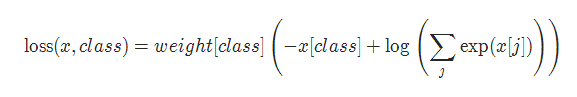torch.nn.CrossEntropyLoss(weight=None,ignore_index=-100, reduction='mean')

weight (Tensor, optional) – 自定义的每个类别的权重. 必须是一个长度为 C 的 Tensor

ignore_index (int, optional) – 设置一个目标值, 该目标值会被忽略, 从而不会影响到 输入的梯度。

reduction-三个值，none: 不使用约简；mean:返回loss和的平均值；sum:返回loss的和。默认：mean。

#### 4 KL 散度损失 KLDivLoss

torch.nn.KLDivLoss(reduction='mean')

reduction-三个值，none: 不使用约简；mean:返回loss和的平均值；sum:返回loss的和。默认：mean。

#### 5 二进制交叉熵损失 BCELoss

torch.nn.BCELoss(weight=None, reduction='mean')

weight (Tensor, optional) – 自定义的每个 batch 元素的 loss 的权重. 必须是一个长度为 “nbatch” 的 的 Tensor

#### 6 BCEWithLogitsLoss

BCEWithLogitsLoss损失函数把 Sigmoid 层集成到了 BCELoss 类中. 该版比用一个简单的 Sigmoid 层和 BCELoss 在数值上更稳定, 因为把这两个操作合并为一个层之后, 可以利用 log-sum-exp 的 技巧来实现数值稳定.

torch.nn.BCEWithLogitsLoss(weight=None, reduction='mean', pos_weight=None)

weight (Tensor, optional) – 自定义的每个 batch 元素的 loss 的权重. 必须是一个长度 为 “nbatch” 的 Tensor

#### 7 MarginRankingLoss

torch.nn.MarginRankingLoss(margin=0.0, reduction='mean')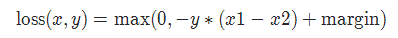margin:默认值0

#### 8 HingeEmbeddingLoss

torch.nn.HingeEmbeddingLoss(margin=1.0,  reduction='mean')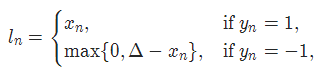margin:默认值1

#### 9 多标签分类损失 MultiLabelMarginLoss

torch.nn.MultiLabelMarginLoss(reduction='mean')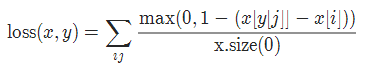#### 10 平滑版L1损失 SmoothL1Loss

torch.nn.SmoothL1Loss(reduction='mean')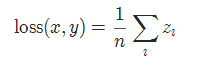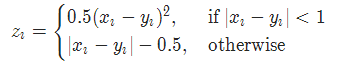#### 11 2分类的logistic损失 SoftMarginLoss

torch.nn.SoftMarginLoss(reduction='mean')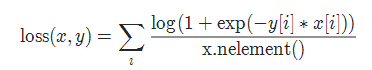#### 12 多标签 one-versus-all 损失 MultiLabelSoftMarginLoss

torch.nn.MultiLabelSoftMarginLoss(weight=None, reduction='mean')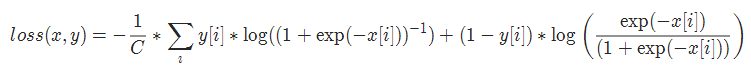#### 13 cosine 损失 CosineEmbeddingLoss

torch.nn.CosineEmbeddingLoss(margin=0.0, reduction='mean')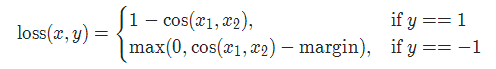margin:默认值0

#### 14 多类别分类的hinge损失 MultiMarginLoss

torch.nn.MultiMarginLoss(p=1, margin=1.0, weight=None,  reduction='mean')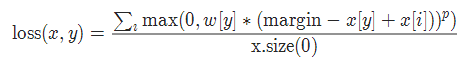p=1或者2 默认值：1
margin:默认值1

#### 15 三元组损失 TripletMarginLoss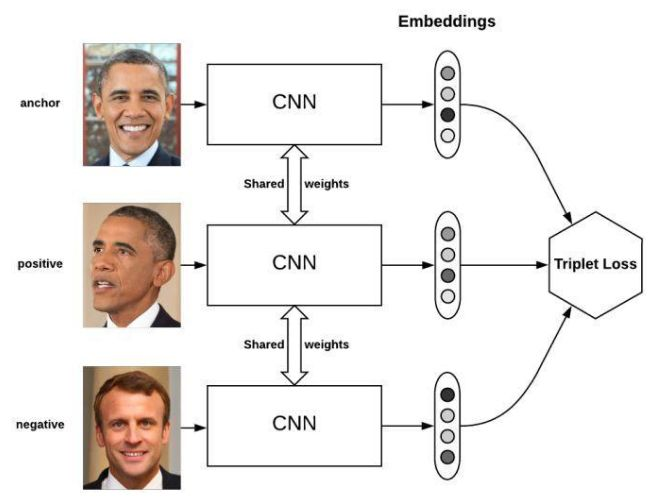torch.nn.TripletMarginLoss(margin=1.0, p=2.0, eps=1e-06, swap=False, reduction='mean')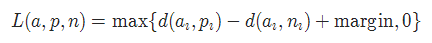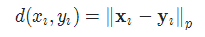#### 16 连接时序分类损失 CTCLoss

CTC连接时序分类损失，可以对没有对齐的数据进行自动对齐，主要用在没有事先对齐的序列化数据训练上。比如语音识别、ocr识别等等。

torch.nn.CTCLoss(blank=0, reduction='mean')

reduction-三个值，none: 不使用约简；mean:返回loss和的平均值；sum:返回loss的和。默认：mean。

#### 17 负对数似然损失 NLLLoss

torch.nn.NLLLoss(weight=None, ignore_index=-100,  reduction='mean')

weight (Tensor, optional) – 自定义的每个类别的权重. 必须是一个长度为 C 的 Tensor

ignore_index (int, optional) – 设置一个目标值, 该目标值会被忽略, 从而不会影响到 输入的梯度.

#### 18 NLLLoss2d

torch.nn.NLLLoss2d(weight=None, ignore_index=-100, reduction='mean')

weight (Tensor, optional) – 自定义的每个类别的权重. 必须是一个长度为 C 的 Tensor

reduction-三个值，none: 不使用约简；mean:返回loss和的平均值；sum:返回loss的和。默认：mean。

#### 19 PoissonNLLLoss

torch.nn.PoissonNLLLoss(log_input=True, full=False,  eps=1e-08,  reduction='mean')

log_input (bool, optional) – 如果设置为 True , loss 将会按照公 式 exp(input) - target * input 来计算, 如果设置为 False , loss 将会按照 input - target * log(input+eps) 计算.

full (bool, optional) – 是否计算全部的 loss, i. e. 加上 Stirling 近似项 target * log(target) - target + 0.5 * log(2 * pi * target).

eps (float, optional) – 默认值: 1e-8

pytorch loss function 总结

http://www.voidcn.com/article/p-rtzqgqkz-bpg.html